# Point

## Resumen

A representation of an x,y pair, optionally with measure, height, and ID attributes.

## Debate

A Point object does not include spatial reference information and is frequently used to construct other geometry objects, including PointGeometry, Polyline, Polygon, and Multipoint objects. In the example below, a Point object is used to create a PointGeometry object.

``````pt = arcpy.Point(-12683890.6, 5811151.5)
pt_geometry = arcpy.PointGeometry(pt, spatial_reference=3857)``````

## Sintaxis

` Point ({X}, {Y}, {Z}, {M}, {ID})`
 Parámetro Explicación Tipo de datos X The X coordinate of the point. (El valor predeterminado es 0.0) Double Y The Y coordinate of the point. (El valor predeterminado es 0.0) Double Z The Z coordinate of the point. (El valor predeterminado es None) Double M The M value of the point. (El valor predeterminado es None) Double ID The shape ID of the point. (El valor predeterminado es 0) Integer

 Propiedad Explicación Tipo de datos ID(Lectura y escritura) An integer used to uniquely identify the point. Integer M(Lectura y escritura) The measure value of the point. Double X(Lectura y escritura) The horizontal coordinate of the point. Double Y(Lectura y escritura) The vertical coordinate of the point. Double Z(Lectura y escritura) The elevation value of the point. Double

## Descripción general del método

 Método Explicación clone (point_object) Clone the Point object. contains (second_geometry, {relation}) Indicates if the base geometry contains the comparison geometry. contains is the opposite of within. Only True relationships are shown in this illustration.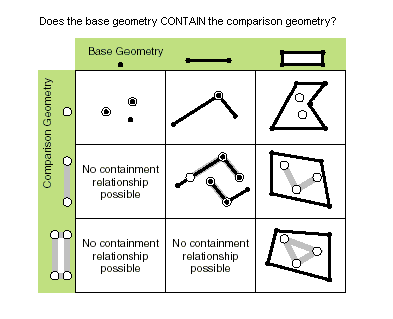crosses (second_geometry) Indicates if the two geometries intersect in a geometry of a lesser shape type. Two polylines cross if they share only points in common, at least one of which is not an endpoint. A polyline and an polygon cross if they share a polyline or a point (for vertical line) in common on the interior of the polygon which is not equivalent to the entire polyline. Only True relationships are shown in this illustration.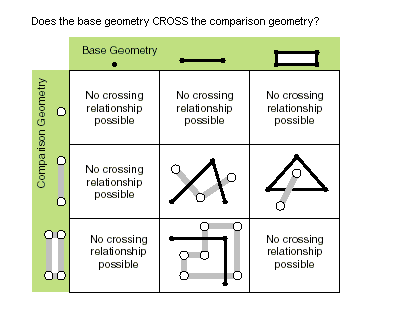disjoint (second_geometry) Indicates if the base and comparison geometries share no points in common. Two geometries intersect if disjoint returns False. Only True relationships are shown in this illustration.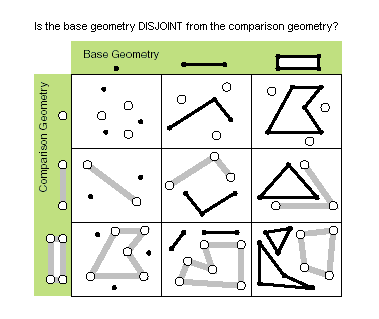equals (second_geometry) Indicates if the base and comparison geometries are of the same shape type and define the same set of points in the plane. This is a 2D comparison only; M and Z values are ignored.Only True relationships are shown in this illustration.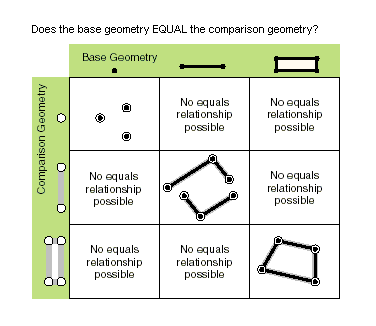overlaps (second_geometry) Indicates if the intersection of the two geometries has the same shape type as one of the input geometries and is not equivalent to either of the input geometries. Only True relationships are shown in this illustration.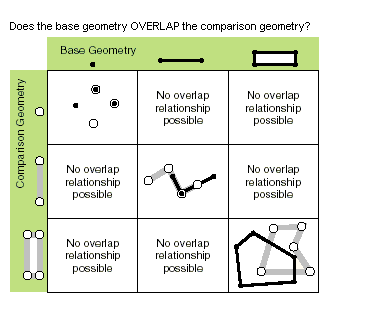touches (second_geometry) Indicates if the boundaries of the geometries intersect. Two geometries touch when the intersection of the geometries is not empty, but the intersection of their interiors is empty. For example, a point touches a polyline only if the point is coincident with one of the polyline end points. Only True relationships are shown in this illustration.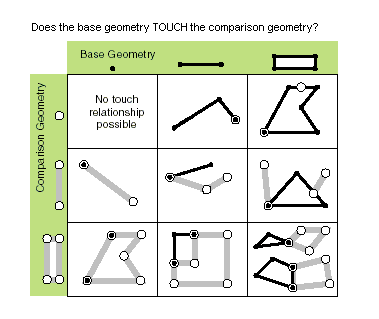within (second_geometry, {relation}) Indicates if the base geometry is within the comparison geometry. within is the opposite operator of contains. Only True relationships are shown in this illustration.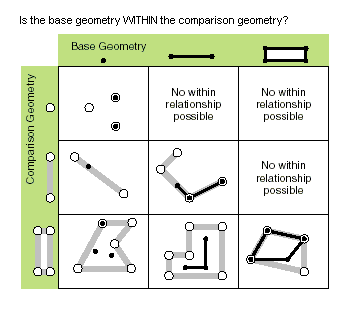The base geometry is within the comparison geometry if the base geometry is the intersection of the geometries and the intersection of their interiors is not empty. within is a Clementini operator, except in the case of an empty base geometry.

## Métodos

`clone (point_object)`
 Parámetro Explicación Tipo de datos point_object A Point object. Point
`contains (second_geometry, {relation})`
 Parámetro Explicación Tipo de datos second_geometry A second geometry. Object relation The spatial relationship type.BOUNDARY— Relationship has no restrictions for interiors or boundaries.CLEMENTINI— Interiors of geometries must intersect. Specifying CLEMENTINI is equivalent to specifying None. This is the default.PROPER— Boundaries of geometries must not intersect.(El valor predeterminado es None) String
Valor de retorno
 Tipo de datos Explicación Boolean A return Boolean value of True indicates this geometry contains the second geometry.
`crosses (second_geometry)`
 Parámetro Explicación Tipo de datos second_geometry A second geometry. Object
Valor de retorno
 Tipo de datos Explicación Boolean A return Boolean value of True indicates the two geometries intersect in a geometry of a lesser shape type.
`disjoint (second_geometry)`
 Parámetro Explicación Tipo de datos second_geometry A second geometry. Object
Valor de retorno
 Tipo de datos Explicación Boolean A return Boolean value of True indicates that the two geometries share no points in common.
`equals (second_geometry)`
 Parámetro Explicación Tipo de datos second_geometry A second geometry. Object
Valor de retorno
 Tipo de datos Explicación Boolean A return Boolean value of True indicates that the two geometries are of the same shape type and define the same set of points in the plane.
`overlaps (second_geometry)`
 Parámetro Explicación Tipo de datos second_geometry A second geometry. Object
Valor de retorno
 Tipo de datos Explicación Boolean A return Boolean value of True indicates the intersection of the two geometries has the same dimension as one of the input geometries.
`touches (second_geometry)`
 Parámetro Explicación Tipo de datos second_geometry A second geometry. Object
Valor de retorno
 Tipo de datos Explicación Boolean A return Boolean value of True indicates the boundaries of the geometries intersect.
`within (second_geometry, {relation})`
 Parámetro Explicación Tipo de datos second_geometry A second geometry. Object relation The spatial relationship type.BOUNDARY— Relationship has no restrictions for interiors or boundaries.CLEMENTINI— Interiors of geometries must intersect. Specifying CLEMENTINI is equivalent to specifying None. This is the default.PROPER— Boundaries of geometries must not intersect.(El valor predeterminado es None) String
Valor de retorno
 Tipo de datos Explicación Boolean A return Boolean value of True indicates this geometry is contained within the second geometry.

## Muestra de código

Point example

Create a Point object and print some of its properties.

``````import arcpy

# Create point object
point = arcpy.Point(2000, 2500)

# Print point properties
print("Point properties:")
print(" X:  {0}".format(point.X))
print(" Y:  {0}".format(point.Y))``````
Point example 2

Examine Point objects returned from a Polygon object.

``````import arcpy

# Create cursor to retrieve Hawaii shape
feature_class = "c:/data/Hawaii.shp"
cursor = arcpy.da.SearchCursor(feature_class, ["SHAPE@"])

for row in cursor:
# Get the geometry object from the shape field
print("Number of Hawaiian islands: {0}".format(row.partCount))

# GetPart returns an array of point objects for each part.
for island in row.getPart():
print("Vertices in island: {0}".format(island.count))
for point in island:
print("X: {0}, Y: {1})".format(point.X, point.Y))``````